Disclosure: This post may contain affiliate links, meaning when you click the links and make a purchase, we receive a commission.

# How to calculate future value with inflation in Excel

Want to know how to calculate the future value with inflation in Excel? Want to calculate inflation-adjusted return from your investment?

You’re in the right place.

Before going into the calculations, I will introduce you with several terms like:

• Inflation
• Future value
• Nominal Interest Rate
• Real Rate of Return

## What is Inflation and how it affects our lives?

The prices of things go up and this is called inflation. Deflation is the antonym of inflation. The prices of things go down in deflation period.

In the following image, we are seeing the inflation and deflation picture of the USA for the last around 100 years.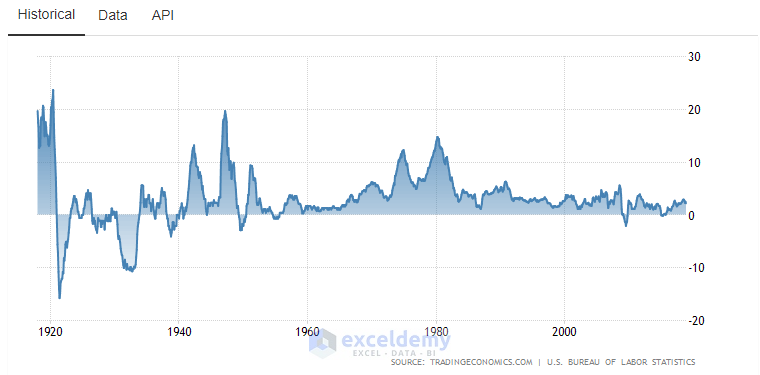From the year 1920 to 1940 (20 years), deflation occurred more than inflation. From there, inflation dominated. So, most of the time, we see the prices of things are going up.

Suppose, you have \$100 cash today. And the projected inflation for the next 1 year is 4%. If you still hold the cash (\$100), after 1 year, your purchase power will be lower (\$96) with that \$100 cash.

If we see the general pricing of things, \$100 product will be priced now at \$104. So, with your holding of \$100 cash, you cannot buy the same product after 1 year that you could buy 1 year before.

So, inflation devalues the cash and increases the price of the product.

This is why holding cash is a bad idea in the investment world.

## Future Value of Money

Future value of money can be thought in two ways:

• The future purchase power of your money. With inflation, the same amount of money will lose its value in the future.
• Return of your money when compounded with annual percentage return. If you invest your money with a fixed annual return, we can calculate the future value of your money with this formula: FV = PV(1+r)^n. Here, FV is the future value, PV is the present value, r is the annual return, and n is the number of years. If you deposit a small amount of money every month, your future value can be calculated using Excel’s FV function. We shall discuss both methods in this tutorial.

## Nominal Interest Rate

If you deposit your money with a bank, the bank provides you interest in your deposits. At the rate, the bank provides your interest is called the Nominal Interest Rate. For example, if your bank provides 6% per year, then the nominal interest rate is 6%.

## Real Rate of Return

You can use this simplified formula to calculate the real rate of return:

Nominal Interest Rate – Inflation Rate = Real Rate of Return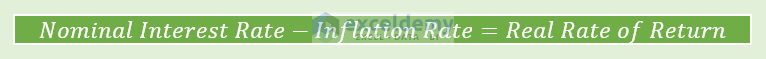To get a Real Rate of Return, you have to deduct the Inflation Rate from the Nominal Interest Rate (or your yearly return).

But the accurate formula is shown below: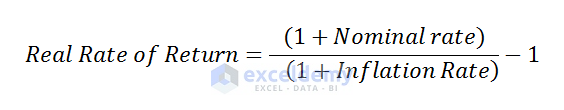Let me explain this concept with an example. Suppose, you have invested \$1000 in the money market and a got 5% return from there. The inflation rate is 3% for this period.

So, your total money is now: \$1000 + \$1000 x 5% = \$1050.

But do your purchase power the same as before? Say, you could buy a product for \$1000, now its price is \$1030 (with 3% inflation).

How many of this product you can buy today?

\$1050/\$1030 = 1.019417476.

So, your REAL purchase power has increased from 1 to 1.019417476.

In % it is: ((1.019417476 – 1)/1)*100% = 0.019417476*100% = 1.9417%

We can reach this percentage also using this formula:

(1.05/1.03)-1 = 1.019417 – 1 = 0.019417 * 100% = 1.9417%

Calculate-future-value-with-inflation-in-Excel

## Calculate future value with inflation in Excel

We shall calculate the future value with inflation in more than one way:

### Example 1: Start with an initial investment and no recurring deposits

You have some investible money and you want to invest the money with the following details:

• Investible money: \$10,000
• Annual return from investment (fixed): 8.5% per year
• Inflation rate (approx.) over the investment time: 3.5%
• Investment period: 10 years

This is the return you will get (following image).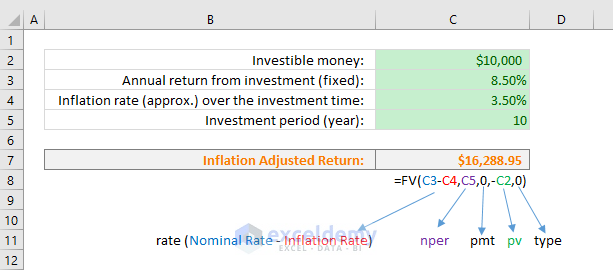Don’t misunderstand one thing. In real life, you will get actually return of amount \$22,609.83 with the following formula (inflation is zero):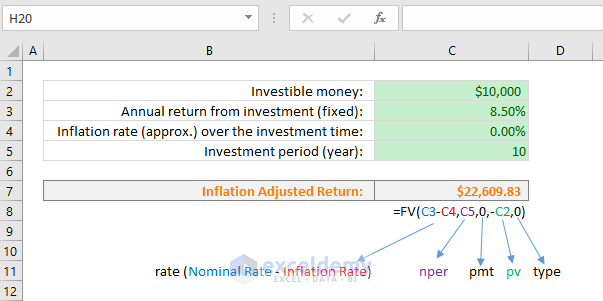You will also come out with the same value if you use the following universal formula. For the value of r, you will use the real rate of return (real rate of return = annual return – inflation rate).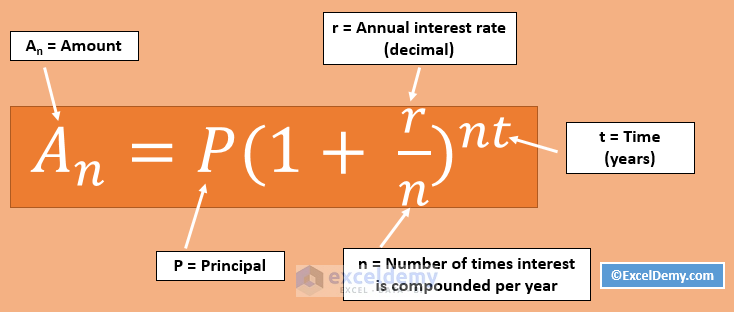### Example 2: Start with an initial investment and make regular deposits

In this example, I am showing a scenario with the following details:

• You’re paying a regular monthly deposit: \$2500
• Interest rate (yearly): 8.5%
• Inflation rate (yearly): 3%
• Payment Frequency/Year: 12
• Total Time (Years): 10
• Payment per Period, pmt: \$2,500.00
• Present Value, PV: 50000
• Payment is done at the beginning of the period

The following image shows the output.Observe that in the cell C7, we have calculated the Interest per Period by subtracting the Yearly Inflation Rate from the Yearly Interest Rate and then divided the value by the Number of Payments per Year.

What if the Yearly Return is lower than the Inflation Rate?

See this image. When annual return is lower than the inflation rate, you will actually lose money.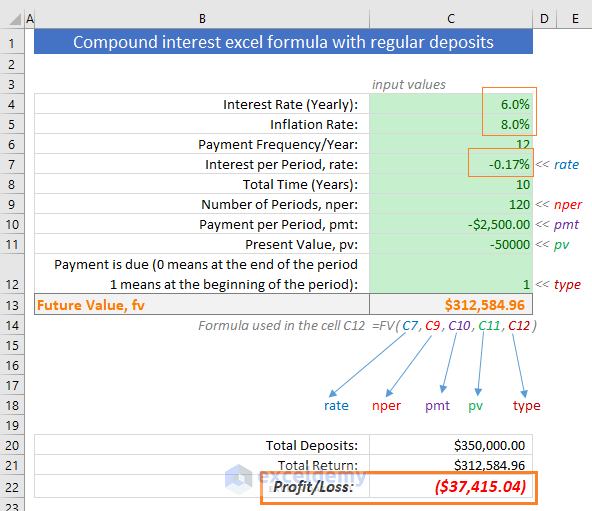## Conclusion

Calculating the future value of your investment is important. But if you are calculating it without considering the Inflation Rate, you’re neglecting a very important thing. If you earn 8% annually from your investment in an economy where the inflation rate is 10%, you’re actually losing the value of your money.

Hello!
Welcome to my Excel blog! It took me some time to be a fan of Excel. But now I am a die-hard fan of MS Excel. I learn new ways of doing things with Excel and share here. Not only how to guide on Excel, but you will get also topics on Finance, Statistics, Data Analysis, and BI. Stay tuned!

1 Comment
1. ReplyPeter Wettig Jul 8, 2020 at 7:08 PM

Hi, I like your website it is very easy to understand (for a simple mind like mine).
There is one example that nobody seems to show (I have been surfing to web for a while trying to find a solution to this problem), may be you might be able to help me please.
Here is the problem as an example;
1. I receive net \$2000 p.m. from rent.
2. I invest this in an investment account that gives me 10% interest p.a.
3. Every year the rental is increase of 2.5% per year
4. Using excel FV calc., FV=(rate=2.083%,nper=120,PMT=?,PV=0,1)
5. The PMT has to be indexed somehow to take into consideration the 2.5% increase in yearly deposits in-line with inflation.
6. I know the answer at the end should be \$453,624.65 (I printed something off a while back but I’ve lost the website) but I cannot come anywhere near this figure.
So, the question is how do I configure this FV formula?
If you can help me, I would be extremely grateful. Thank you in advance.